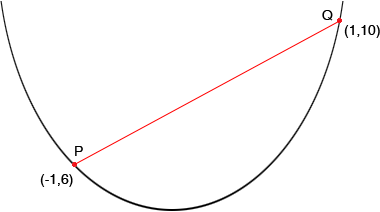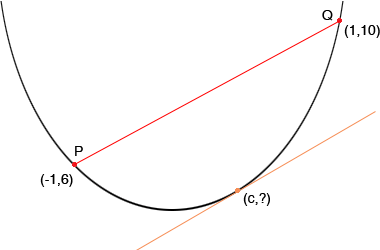Hi My name is Candace and I am a secondary student who is having a lot of trouble with mean value theorem. One of the questions looks like this: Verify that the function satisfies the hypotheses of the Mean Value Theorem on the given interval. Find all numbers "c" that satisfy the Mean Value Theorem. 11. f(x)=3x2 + 2x +5 [-1, 1] If you could help me understand what to do and why that would be great. Hi Candace, I expect that in your textbook the conditions given for the Mean Value Theorem are either that "f(x) is differentiable for all x in [a,b]" or that "f(x) is differentiable for all x in (a,b) and continuous for all a in [a,b]". The function f(x)=3x2 + 2x +5 is differentiable for all values of x and hence it satisfies the conditions for any interval [a,b] and hence in particular for [-1,1]. f(1) = 312 + 21 + 5 = 10 and f(-1) = 3(-1)2 + 2(-1) + 5 = 6. I drew a rough sketch of the graph of f(x) = 3x2 + 2x +5 and put the points P(-1,6) and Q(1,10) on the graph.The conclusion of the Mean Value Theorem is that somewhere on the curve between P and Q there is a point where the tangent line is parallel to the line joining P and Q. The first coordinate of this point is the number "c" in your problem. Before I try to find c using calculus let me put it on the curve where I think it will be. I just tried to find a point where it looks like the tangent line is parallel to the line joining P and Q. I used ? for the second coordinate of this point since I don't care what it is, I just want to find c.Algebraically I can tell if the two lines are parallel by calculating their slopes. I know the coordinates of P and Q so the slope of the line joining P and Q is  (10 - 6)/(1 - (-1))  = 4/2 = 2 The second line is the tangent to the curve at the point (c,?) so its slope is f '(c). f '(x) = 6x + 2 and hence the slope of the tangent at (c,?) is f '(c) = 6c + 2. Thus the two lines are parallel if 6c + 2 = 2 that is 6x = 0 or c = 0. Your textbook probably has the conclusion that f '(c) = (f(b) - f(a))/(b - a)  But that is exactly what we did. f '(c) = 6c - 2 and  (f(b) - f(a))/(b - a)  =  (10 - 6)/(1 - (-1)) =2 Penny